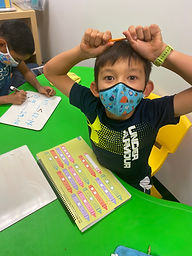## Ms. Briana

### Target 1​

###### Lesson Type:

Review

Number Operation

:

Computation

Add within 1,000 (regrouping limited to only one place-value).

###### 1:

Understand that the same process is utilized to add three or more whole numbers that is used to add only two numbers.

###### 2:

Understand how to regroup based on place-value understandings (i.e., ten ones can be composed into one ten – ten tens can be composed into one hundred).

###### 3:

Understand why the standard algorithm for adding is based on place-value.

###### 4:

Understand that when adding a multi-digit number, to solve start in the lowest place-value and go left.

###### 5:

Understand that when adding a multi-digit number, each place-value is being added individually to create the new sum (ones are added to ones, tens are added to tens, etc.)

1st

###### Vocabulary:

Multi-digit, Place Value, Regrouping and Sum

Activities:

Students rolled dice to create addition equations.

Students usd workbooks to practice multi-digit addition.### Home Exploration

###### Guiding Questions:## Absent Students:

Sid

### Target 2

:

2nd

###### Vocabulary:

Compare, Quantities, Symbols, Greater than, Lesser Than and Equal to

Activities:

Students used Multisided dice to create addition and subtraction equations for them to compare.

Students created equations comparisons for their peers to solve.

Students practiced in their workbooks.### Home Exploration

###### Guiding Questions:### Target 3

:

###### 1:

Define an equal sign as a symbol which shows that the quantity on both sides are the same.

###### 2:

Understand that an equal sign can link an equation to its solution or that it can link two equations together.

2nd

###### Vocabulary:

Activities:

Students were given an equal sign and they were asked to discribe what it means.

Students were then given equivalent equations for them to identify as true or untrue. If untrue they have to figure out how to make it true.

Students used playing cards to create 1 full equation and 1 partial equation.

Students rolled dice and created partial equations for thier partner to finish and make equivalent.### Home Exploration